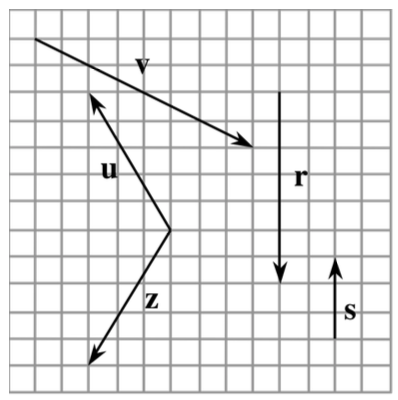### Home > PC > Chapter 10 > Lesson 10.1.2 > Problem10-23

10-23.

On graph paper, copy the vectors shown at right. Draw and label each of the following vectors. Leave room to separate each answer from the others.

1. $\textbf{p}$, a vector equivalent to $\textbf{v}$

2. $\textbf{u} + \textbf{z}$

3. $\frac { 1 } { 2 }\textbf{v}$

4. $\textbf{u} − \textbf{v}$